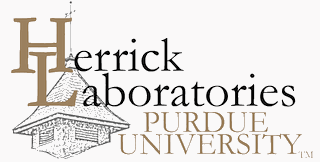## International Refrigeration and Air Conditioning Conference

2157

2012

#### Keywords

Absorption Heat Pump, Thermophysical Properties, Lithium Bromide, 1, 2-Propanediol

#### Abstract

The solubilities, densities and viscosities of lithium bromide (LiBr) + 1, 2-propanediol (HO-CH2-CHOH-CH3) aqueous solution (mass ratio of LiBr/HO-CH2-CHOH-CH3 = 3.5, 4.5 and 5.5) were measured in the mass fraction range from 0.30 to 0.75. Solubility measurements were performed by the visual method in the temperature range of (271.15 to 345.15) K. The density measurements were made using an automated vibrating tube density meter, and the viscosity measurements were carried out with an automated falling-ball viscometer in the temperature range of (293.15 to 363.15) K. The density and viscosity data were correlated with appropriate regression equations as a function of the mass fraction and temperature. The maximum average absolute deviations (AAD) between experimental and correlated data were 0.08% and 1.51% for densities and viscosities, respectively.

COinS